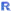# Category:Regression

(Redirected from Regression)
Related Online Training modules
Regression
Generally it is best to access online training from within Q by selecting Help : Online Training

Q's options for doing regression and driver analysis are contained in the Create > Regression section of the menu. This page summarizes the different options that are available. The most powerful options for regression are those which use Standard R, and these are marked with theicon. These include linear regression, as well as various types of logistic and Poisson regression. Many of these regression items can also perform a kind of driver analysis which is called Relative Importance Analysis.

Additional driver analysis options are available under Create > Regression > Driver (Importance) Analysis, which includes Shapley driver analysis.

Diagnostics are available for the Standard R () regressions, and these are contained in Create > Regression > Diagnostic. Options for saving new variables to your data set based on the Standard R regression outputs can be found in Create > Regression > Save Variable(s). You should always select the regression item from your report before running the diagnostic options and the options for saving new variables.

While the options discussed above are generally better, the menu also includes the Legacy Regression option. This option used to be called Create > Traditional Multivariate Analysis > Regression in older versions of Q.

## Standard R Regression Options

The Standard R () regression features all share the same interface and options, and you can even switch between regression types where necessary. The menu items are:

Additionally, any of these models can be run in a stepwise mode by first using one of the options above, and then using:

## Saving Variables

The options in Create > Regression > Save Variable(s) allow you to save out new variables from any of the Standard Rregression options listed above. To save variables from a regression, first select the output of the regression in the Report, and then select one of the following items:

• Fitted Values - Fit values for the outcome variable from the regression model for each case that was used in the estimation (except cases with missing data).
• Predicted Values - Save predicted values for the outcome variable for all cases in the sample (except cases with missing data).
• Probabilities of Each Response - For regression models with discrete (categorical) outcome variables, save a new variable for each category in the outcome which gives the probability that each case is predicted to be in that category. Cases with missing data are not included.
• Residuals - Create a new variable which computes the difference between the predicted value and the observed value for each case. Cases with missing data are not included.

New R Variables will be saved to your project which contain the results of the calculations based on the regression model that you had selected. If you make changes to the regression output, the new variables will be updated to reflect the changes.

## Diagnostic Options

The options in Create > Regression > Diagnostic create additional outputs that can assist with diagnosing issues in the Standard Rregression options listed above.

## Driver Analysis

The features in Create > Regression > Driver (Importance) Analysis use QScript to generate special tables which display the results of different kinds of driver analysis. These tables can be filtered and weighted just like normal tables in Q, and you may even add a second question to the Brown drop-down to estimate the driver analysis separately for different sub-groups. Each of these driver analysis features exclude respondents who have missing data in any of the input variables. Details about the different driver analysis features is on the page Driver (Importance) Analysis.

## Older Versions of Q

Legacy Regression Regression where the blue drop-down question is the dependent variable.